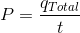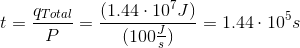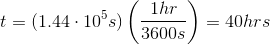# AP Chemistry : Laws of Thermodynamics

## Example Questions

### Example Question #1 : Laws Of Thermodynamics

Which of the following is a law of thermodynamics?

ΔS (system) + ΔS (surroundings) = ΔS (universe)

ΔE (surroundings) = ΔE (system)

The entropy of the universe is always decreasing

ΔH (system) + ΔH (surroundings) = ΔH (universe)

ΔS (system) + ΔS (surroundings) = ΔS (universe)

Explanation:

The second law of thermodynamics states that the entropy of the system and the entropy of the surroundings is equal to the entropy of the universe. The rest of the answer choices are not one of the fundamental laws of themodynamics.

### Example Question #2 : Laws Of Thermodynamics

Which of the following is true regarding the first law of thermodynamics?

It states that the heat lost by a system is gained by the surroundings

Conversion of kinetic energy to potential energy violates the first law of thermodynamics

An exothermic reaction always destroys energy, whereas an endothermic reaction always creates energy

It states that the disorder in the universe is always increasing

It states that the heat lost by a system is gained by the surroundings

Explanation:

First law of thermodynamics states that the energy of the universe is always constant. This is called the conservation of energy. The total energy of the universe is defined as follows: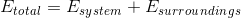According to the law, energy gained or lost by a system is reciprocated by its surroundings. This means that energy, such as heat energy, lost by the system will be gained by the surroundings. This will keep the total energy constant. Energy can be converted from one form to another (such as from kinetic to potential); however, it cannot be created or destroyed.

The second law of thermodynamics states that the disorder of the universe is always increasing. As mentioned, energy can never be created, nor destroyed. In an exothermic reaction, heat energy is transferred from the system to the surroundings. In an endothermic reaction, heat energy is transferred from the surroundings to the system.

### Example Question #2 : Laws Of Thermodynamics

Which of the following substances will have the lowest entropy?

Liquid water

Water vapor

Product of a vaporization reaction

Product of a freezing reaction

Product of a freezing reaction

Explanation:

Entropy is a measure of disorder; therefore, a substance with low entropy will be highly ordered. Gases have the highest entropy because the molecules are spread far apart and are more chaotic. Solids, on the other hand, have the least entropy because they are typically found in an ordered, lattice structure with very little chaos. A freezing reaction involves conversion of a liquid to a solid; therefore, the product of this reaction will have the lowest entropy.

### Example Question #4 : Laws Of Thermodynamics

A researcher creates a perfect crystal and stores it at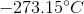. What can you conclude about this substance?

I. The entropy of this substance is less than zero

II. It will have more microstates than gaseous nitrogen

III. Its Gibbs free energy is equal to its enthalpy

I and III

I only

III only

II and III

III only

Explanation:

The third law of thermodynamics states that a perfect crystal at absolute zero (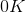or) has an entropy of zero (lowest possible entropy). Note that entropy can never be less than zero.

Microstates are microscopic configurations of a substance, and relates directly to the entropy of the system. The more microstates, the higher the entropy. Since this substance has the lowest entropy it will have the least amount of microstates.

To understand the relationship between Gibbs free energy and enthalpy, we need to look at the following equation: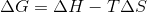Here,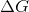is change in Gibbs free energy,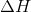is enthalpy,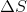is change in entropy, and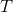is the temperature in Kelvin. From the given information, we know that both temperature and entropy are zero; therefore, Gibbs free energy equals the enthalpy for this substance.

### Example Question #5 : Laws Of Thermodynamics

A chemistry student is trying to calculate how long it will take a power source of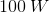to heat a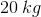sample of ice from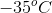to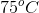. Given that the specific heat capacity of ice is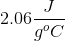, the specific heat capacity of liquid water is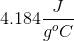, and the heat of fusion for water is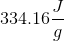, how long will this process take?

There is not enough information to determine the amount of time needed for the process described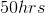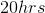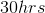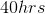Explanation:

In order to solve this problem, we'll need to break it up into steps.

Step 1: Calculate the amount of energy necessary to raise the sample of ice fromto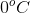. To do this, we'll need to use the following equation: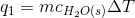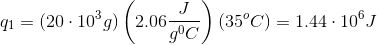Step 2: Calculate the amount of energy necessary to convert the sample atfrom ice to water. We'll need to make use of the following equation: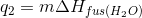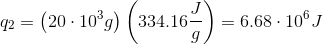Step 3: Calculate the amount of energy necessary to convert the sample of water fromto.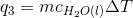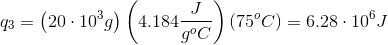Step 4: Sum the amount of energy from the previous 3 steps. This value is the total amount of energy for the entire process.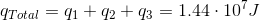Step 5: Now that we know the total amount of energy needed for the process, we need to calculate the time based on the amount of power provided.